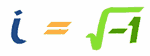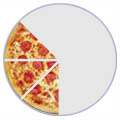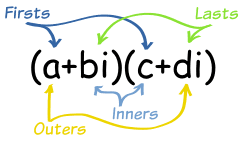# 复数实数是像这样的数：

 1 12.38 −0.8625 3/4 √2 1998虚数平方数。

• 正数的平方是正数，
• 负数的平方也是正数（因为负负得正），例如 −2 × −2 = +4i2 = −1

 3i 1.04i −2.8i 3i/4 (√2)i 1998i

## 复数### 例子：

 1 + i 39 + 3i 0.8 − 2.2i −2 + πi √2 + i/2

### 一个数可以是两个数的组合吗？3 + 2i 3 2
5 5 0
−6i 0 −6

## 复杂吗？## 视觉解释## 加法

(a+bi) + (c+di) = (a+c) + (b+d)i

### 例子：3 + 2i 加 1 + 7i

• 加实部，
• 加虚部：

(3 + 2i) + (1 + 7i)
= 3 + 1 + (2 + 3)i
= (4 + 9i)

### 例子：3 + 5i 加 4 − 3i(3 + 5i) + (4 − 3i)
= 3 + 4 + (5 − 3)i
= 7 + 2i

## 乘法首：a × c 外：a × di 内：bi × c 尾：bi × di (a+bi)(c+di) = ac + adi + bci + bdi2

### 例子：(3 + 2i)(1 + 7i)

 (3 + 2i)(1 + 7i) = 3×1 + 3×7i + 2i×1+ 2i×7i = 3 + 21i + 2i + 14i2 = 3 + 21i + 2i − 14 （因为 i2 = −1） = −11 + 23i

### 例子： (1 + i)2

 (1 + i)2 = (1 + i)(1 + i) = 1×1 + 1×i + 1×i + i2 = 1 + 2i − 1 （因为 i2 = −1） = 0 + 2i

### 公式背后……

 (a+bi)(c+di) = ac + adi + bci + bdi2 首外内尾法 = ac + adi + bci − bd （应为 i2 = −1） = (ac − bd) + (ad + bc)i （拼合同类项)

### 例子：i2

i 可以写成实部与虚部的组合：0 + i

 i2 = (0 + i)2 = (0 + i)(0 + i) = (0×0 − 1×1) + (0×1 + 1×0)i = −1 + 0i = −1

## 共轭### 例子：

5 − 3i   =   5 + 3i

## 除法

### 例子：

 2 + 3i 4 − 5i

 2 + 3i × 4 + 5i = 8 + 10i + 12i + 15i2 4 − 5i 4 + 5i 16 + 20i − 20i − 25i2

 = 8 + 10i + 12i − 15 16 + 20i − 20i + 25

 = −7 + 22i 41

 = −7 + 22 i 41 41

## 乘以共轭

(4 − 5i)(4 + 5i) = 16 + 20i − 20i − 25i2

(4 − 5i)(4 + 5i) = 42 + 52

(a + bi)(a − bi) = a2 + b2

### 例子：再做一遍

 2 + 3i 4 − 5i

2 + 3i × 4 + 5i   =   8 + 10i + 12i + 15i2
4 − 5i 4 + 5i 16 + 25

=
 −7 + 22i 41

 = −7 + 22 i 41 41

## 曼德勃罗集美丽的曼德勃罗集（见图）是基于复数的. 曼德勃罗集是把这个简单的方程式 z2+c（两个变量都是复数）的结果重复地代回 z 里的图。 颜色显示 z2+c 增长得多快，黑色表示它的值停留在一个范围内。 这是把曼德勃罗集放大后的图像这是上图的中间，再放大：